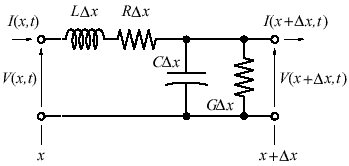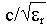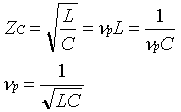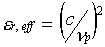HOMETHIN FILM MICROSTRIP TRANSMISSION LINES

 APPLICATION NOTE USM 104 Author: Mihaela Radulescu, M Physic. Sc. member technical staff, SEMICONIX CORPORATION Microstrip lines are widely used in the microwave integrated circuits from hybrids to monolithics.The microstrip line is composed of a thin conducting metal strip of width Wo, thickness t and length L placed on a nonmagnetic dielectric substrate that is in turn placed on a conducting metal ground-plane. The substrate has thickness T and relative permittivity er. The structure is shown in figure 1. Above the conducting strip is a second dielectric material, air.Fig.1 Microstrip line structure Zo, the characteristic impedance, t, the propagation delay that depends on the length of the line, and a, the attenuation constant, are the parameters used to model the transmission line. All physical transmission media have some loss. Considering a small segment of a lossy transmission line distributed circuit model as show in figure 2, the losses in the transmission media are depicted by the series and the shunt resistors. These resistors represent the finite conductivity of the conductors and the dielectric insulator between the conductors respectively. The constants R, G, L, and C are defined as per unit length circuit parameters. The total length of the transmission line segment is Dx.Fig.2 Distributed circuit model of a lossy transmission line. The advantages of using the microstrip line is that it is well suited for the printed circuit fabrication process, and because the strip conductor is exposed on the top side, component mounting is relatively easy. However, since the dielectric around the conductor is inhomogeneous, EM fields can not propagate in TEM mode. This is because a TEM wave must have a velocity c in the air above the strip and one ofin the substrate below. Because of the different velocities needed for TEM propagation, it is not possible to form a single TEM mode. Consequently, small amount of transverse fields must exist to equalize the propagation velocities in different dielectrics. This hybrid presence of TE and TM modes form the principle mode of propagation in a microstrip line which is named quasi-TEM mode. At low frequencies, up to a few GHz, static analysis and TEM mode formulae can be used to approximate the quasi-TEM transmission line characteristics. At frequencies above that, dynamic analysis must be used to solve for all fields components in order to characterize the microstrip line. For the microstrip line in Fig. 1, the propagation velocity np and characteristic impedance Zc can be approximated at low frequencies by applying the TEM mode equations 1 and 2 which are restated below.If we remove the dielectric substrate under the strip and fill it with air , the transmission line is surrounded by homogeneous dielectric and true TEM mode can propagate on the line.where c is the speed of light in air. Since we assumed the dielectric substrate in the microstrip line is nonmagnetic, i.e. µr=1, the per unit length inductance L remains the same and one gets : The term C/Co is called the effective relative permittivity of the microstrip line er,eff . Both C and CO can be found by solving the static field equations for this structure . However, oncer,eff  is calculated from the static capacitances, the microstrip line can be approximated by a TEM strip line surrounded homogeneously with dielectric whose relative permittivity er,eff. In dynamic analysis, although static capacitances are not defined er,eff  is still being used by solving for phase velocity in the quasi-TEM mode , i.e. eq.7:If the width Wo of the microstrip line is changed smaller, both C and Co decrease. Consequently, Zc becomes higher. Likewise, widening the line width reduces its characteristic impedance. The effective relative permittivity also changes with line width but not as obvious because of the ratio. In the case of a very wide line almost all electric field lines are packed between the strip and the ground plane with or without the substrate. Therefore, the ratio C/Co is very close to the relative permittivity of the substrate er, but can never quite become that. As the width of the line shrinks, proportionally more and more field lines reach the air space above the substrate. As a result, the ratio C/Co drops, but it can never reach 1. So, the effective relative permittivity of the microstrip line increases as the width of the line increases, decreases as the width of the line decreases. However, its value will always be in between the relative permittivity of the substrate and the air, i.e. 1
 US MICROWAVES www.usmicrowaves.com Tel:(408)986-8026 Fax:(408)986-8027